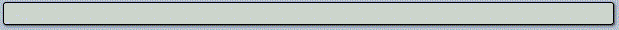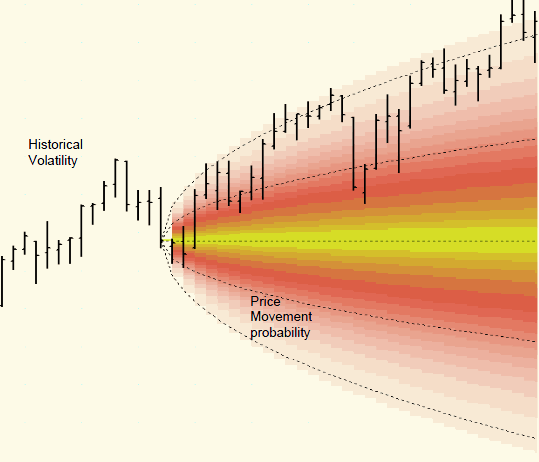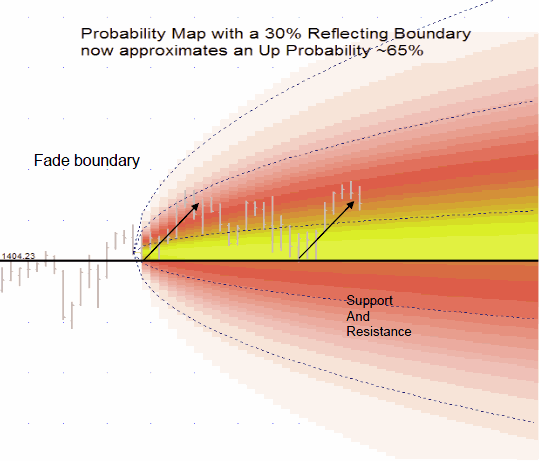Our Technology | Feedback | Scale Invariance | Spatial Probability | Neural Networks | Validation

## Spatial Probability Mapping

Normal diffusion laws can be used to predict the probability of finding a stock at a certain price N steps into the future if price movement follows a random walk. Random walk assumptions do NOT predict that support or resistance lines will have any effect. Parallax has been testing this assumption by isolating and testing price action following contact with support or resistance lines. We seek to find out how much reality deviates from random assumptions and then map these deviations. The first figure shows a random diffusion pattern with curves at -2, -1, 0, 1 and 2 standard deviations.

####Initial statistical tests done on horizontal support and resistance lines revealed a 65% probability of price being found above support (below resistance) when price starts out above and nearby. The following figure shows the probability map with this boundary correction.

####Parallax is in the process of extending our research to channel lines, which may be sloped at any angle. The following figure shows what a probability map might look like with a reflecting support line that is also attracting price toward it.